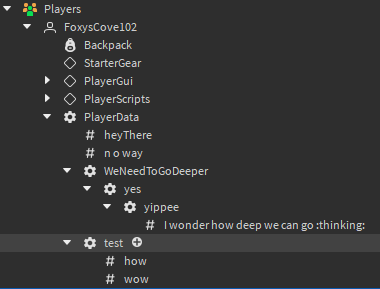# Help Creating Save Tables

Hello,

I am working on the datastore for my game, and I am trying to figure out how to load a huge system of tables, create instances of them in a folder in the player, go through all instances in folder, and then save all the data. Here is what I have so far:

``````local http = game:GetService("HttpService")
local ds = game:GetService("DataStoreService")
local database = ds:GetDataStore("test-database")
local ToSave = {
["heyThere"] = {"abcdefg","StringValue"},
["n o way"] = {72,"NumberValue"},
["test"] = {{
["wow"] = {"zers","StringValue"},
["how"] = {5,"IntValue"}
},"table"},
["WeNeedToGoDeeper"] = {{
["yes"] = {{
["yippee"] = {{
["I wonder how deep we can go :thinking:"] = {34,"IntValue"}
},"table"}
},"table"}
},"table"}
}

local function constructPlayerData(player)
local pdata = Instance.new("Configuration")
pdata.Name = "PlayerData"
pdata.Parent = player
local function createTable(ptable, pparent)
for index, value in pairs(ptable) do
print(index)
print(value)
if value ~= "table" then
local newval = Instance.new(value)
newval.Name = index
newval.Value = value -- This is just the default
newval.Parent = pparent
else
local newFolder = Instance.new("Configuration")
newFolder.Name = index
newFolder.Parent = pparent
createTable(value, newFolder)
end
end
end
createTable(ToSave, pdata)
end

local function deconstructPlayerData(player)
local pdata = player:FindFirstChild("PlayerData")
local TBSaved = {}
local function deconstructTable(ptable, pparent)
for _, instance in pairs(ptable:GetDescendants()) do
if instance:IsA("Folder") then
TBSaved[instance.Name] = {instance.Value, type(instance)}
else
TBSaved[instance.Name] = {thisIsWhereIAmStuck, "table"}
deconstructTable(instance, pparent)
end
end
end
deconstructTable(pdata, pdata)
end

game.Players.PlayerRemoving:Connect(deconstructPlayerData)
``````

Image of the creation working:The part that I am struggling with is where the undefined variable `thisIsWhereIAmStuck` is. Does anyone know how I would go about doing what I want?

Best regards,
– Ham

I went through the table you provided and created a function that I believe should do what you are looking for.
```local function deconstructPlayerData(player) local pdata = player:FindFirstChild(“PlayerData”) local TBSaved = {} local function deconstructTable(ptable, pparent) for _, instance in pairs(ptable:GetDescendants()) do if instance:IsA(“Folder”) then TBSaved[instance.Name] = {instance.Value, type(instance)} else TBSaved[instance.Name] = {thisIsWhereIAmStuck, “table”} deconstructTable(instance, pparent) end end end deconstructTable(pdata, pdata)```

``` for index, value in pairs(TBSaved) do if value == "table" then print(index .. " is a table") else print(index .. " is not a table") end end ```

```end ```
The for loop at the end of the function will print whether or not the table is a table.

Hello,

Thank you for your response. Unfortunately, after reviewing the code you provided, I cannot see how this answered any of my questions. I said that I was having trouble with where the variable `thisIsWhereIAmStuck` is, yet you didn’t change anything on that line. I’m not trying to figure out what values are tables or not, I can already do that.

Thanks,
– Ham

Do you mean an offense sir or ma’am?

I think I have solved your issue, I’m unable to explain it since its too hard for me to explain lol, but heres you long awaited code:

``````local http = game:GetService('HttpService')
local ds = game:GetService('DataStoreService')
local database = ds:GetDataStore('test-database')
local ToSave = {
['heyThere'] = {'abcdefg','StringValue'},
['n o way'] = {72,'NumberValue'},
['test'] = {{
['wow'] = {'zers','StringValue'},
['how'] = {5,'IntValue'}
},'table'},
['WeNeedToGoDeeper'] = {{
['yes'] = {{
['yippee'] = {{
['I wonder how deep we can go :thinking:'] = {34,'IntValue'}
},'table'}
},'table'}
},'table'}
}

-- Functions
function CreateInstance(instanceType: string, parent: Instance, properties: { string: any })
local function SetValue(object: Instance, property: string, value: any)
object[property] = value
end

local object = Instance.new(instanceType, parent)

for property, value in pairs(properties) do
local pass, err = pcall(SetValue, object, property, value)
if not pass then warn(err) end
end

return object
end

function DeserializeData(data, name: string, location: Instance): Instance
local deserializedFolder = CreateInstance('Configuration', location, { Name = name })

local function Create(list, parent: Instance)
--// Types
local types = {
['table'] = function(index: string, value: { any })
print(parent)
Create(
value,
CreateInstance('Configuration', parent, { Name = index })
)
end,
}

--// Main Loop
for index, value in pairs(list) do
local first, second = value, value
local type = types[ second ]

if type then
type(index, value)
continue
end

CreateInstance(second, parent, { Name = index, Value = first })
end
end

Create(data, deserializedFolder)

return deserializedFolder
end

function SerializeData(deserializedData: Instance): { any }
local serializedData = {}

local function Serialize(parent: Instance, list: {})
for _, child: Instance in pairs(parent:GetChildren()) do
if table.find({ 'Configuration', 'Folder' }, child.ClassName) then
list[child.Name] = {}
Serialize(child, list[child.Name])
table.insert(list[child.Name], 2, 'table')

continue
end

if not child:IsA('ValueBase') then continue end

list[child.Name] = { child.Value, child.ClassName }
end
end

Serialize(deserializedData, serializedData)

return serializedData
end

-- Events